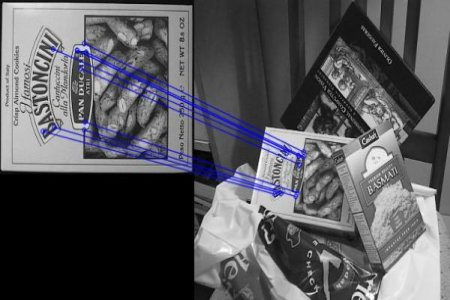# OpenCV-Python 系列 四十三 | 特征匹配

### 目标

– 我们将看到如何将一个图像中的特征与其他图像进行匹配。
– 我们将在OpenCV中使用Brute-Force匹配器和FLANN匹配器

### Brute-Force匹配器的基础

#### 使用ORB描述符进行Brute-Force匹配

import numpy as np
import cv2 as cv
import matplotlib.pyplot as plt
# 初始化ORB检测器
orb = cv.ORB_create()
# 基于ORB找到关键点和检测器
kp1, des1 = orb.detectAndCompute(img1,None)
kp2, des2 = orb.detectAndCompute(img2,None)


# 创建BF匹配器的对象
bf = cv.BFMatcher(cv.NORM_HAMMING, crossCheck=True) # 匹配描述符.
matches = bf.match(des1,des2) # 根据距离排序
matches = sorted(matches, key = lambda x:x.distance) # 绘制前10的匹配项
img3 = cv.drawMatches(img1,kp1,img2,kp2,matches[:10],None,flags=cv.DrawMatchesFlags_NOT_DRAW_SINGLE_POINTS) plt.imshow(img3),plt.show()#### 什么是Matcher对象？

matchs = bf.match(des1,des2)行的结果是DMatch对象的列表。该DMatch对象具有以下属性：
– DMatch.distance-描述符之间的距离。越低越好。
– DMatch.trainIdx-火车描述符中的描述符索引
– DMatch.queryIdx-查询描述符中的描述符索引
– DMatch.imgIdx-火车图像的索引。

#### 带有SIFT描述符和比例测试的Brute-Force匹配

import numpy as np
import cv2 as cv
import matplotlib.pyplot as plt
# 初始化SIFT描述符
sift = cv.xfeatures2d.SIFT_create()
# 基于SIFT找到关键点和描述符
kp1, des1 = sift.detectAndCompute(img1,None)
kp2, des2 = sift.detectAndCompute(img2,None)
# 默认参数初始化BF匹配器
bf = cv.BFMatcher()
matches = bf.knnMatch(des1,des2,k=2)
# 应用比例测试
good = []
for m,n in matches:
if m.distance < 0.75*n.distance:
good.append([m])
# cv.drawMatchesKnn将列表作为匹配项。
img3 = cv.drawMatchesKnn(img1,kp1,img2,kp2,good,None,flags=cv.DrawMatchesFlags_NOT_DRAW_SINGLE_POINTS)
plt.imshow(img3),plt.show()#### 基于匹配器的FLANN

FLANN是近似最近邻的快速库。它包含一组算法，这些算法针对大型数据集中的快速最近邻搜索和高维特征进行了优化。对于大型数据集，它的运行速度比BFMatcher快。我们将看到第二个基于FLANN的匹配器示例。

FLANN_INDEX_KDTREE = 1
index_params = dict(algorithm = FLANN_INDEX_KDTREE, trees = 5)


FLANN_INDEX_LSH = 6
index_params= dict(algorithm = FLANN_INDEX_LSH,
table_number = 6, # 12
key_size = 12,     # 20
multi_probe_level = 1) #2


import numpy as np
import cv2 as cv
import matplotlib.pyplot as plt
# 初始化SIFT描述符
sift = cv.xfeatures2d.SIFT_create()
# 基于SIFT找到关键点和描述符
kp1, des1 = sift.detectAndCompute(img1,None)
kp2, des2 = sift.detectAndCompute(img2,None)
# FLANN的参数
FLANN_INDEX_KDTREE = 1
index_params = dict(algorithm = FLANN_INDEX_KDTREE, trees = 5)
search_params = dict(checks=50)   # 或传递一个空字典
flann = cv.FlannBasedMatcher(index_params,search_params)
matches = flann.knnMatch(des1,des2,k=2)
# 只需要绘制好匹配项，因此创建一个掩码
matchesMask = [[0,0] for i in range(len(matches))]
# 根据Lowe的论文进行比例测试
for i,(m,n) in enumerate(matches):
if m.distance < 0.7*n.distance:
draw_params = dict(matchColor = (0,255,0),
singlePointColor = (255,0,0),
flags = cv.DrawMatchesFlags_DEFAULT)
img3 = cv.drawMatchesKnn(img1,kp1,img2,kp2,matches,None,**draw_params)
plt.imshow(img3,),plt.show()